International Journal of Materials Science and Applications
Volume 5, Issue 6-1, December 2016, Pages: 11-15

Matlab / Simulink Based Simulation of Monocrystalline Silicon Solar Cells

B. Zaidi1, I. Saouane2, M. V. Madhava Rao3, R. Li4, B. Hadjoudja1, S. Gagui1, B. Chouial1, A. Chibani1

1Laboratoire Des Semi-Conducteurs, Département De Physique, Faculté Des Sciences, Université Badji-Mokhtar, Annaba, Algérie

2Laboratoire De Physique Énergétique, Faculté Des Sciences, Université Constantine,  Constantine, Algérie

3Department of Physics, Osmania University, Hyderabad, India

4Department of Chemical Engineering, Louisiana State University, LA, USA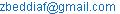(B. Zaidi)

B. Zaidi, I. Saouane, M. V. Madhava Rao, R. Li, B. Hadjoudja,  S. Gagui, B. Chouial, A. Chibani. Matlab / Simulink Based Simulation of Monocrystalline Silicon Solar Cells. International Journal of Materials Science and Applications. Special Issue: Energy and Materials. Vol. 5, No. 6-1, 2016, pp. 11-15. doi: 10.11648/j.ijmsa.s.2016050601.13

Received: June 27, 2016; Accepted: June 29, 2016; Published: September 3, 2016

Abstract: A solar cell is an electrical device that converts light into an electric current. Electron-hole pairs are generated inside the solar cell when light is absorbed. A two diodes equivalent model is used to describe the electronic properties of solar cells. The open-circuit voltage (VOC) is created by the series resistance and the shunt resistant of the solar cell. The theme of modeling is based on the solar radiation, temperature of system and environment to determine the effect of cell parameters like photo generated current, saturation current, series resistance, shunt resistance and ideality factor on the performance of the solar cell. In this paper we study the influence of temperature, series resistance and shunt resistance on the current-voltage (I-V) characteristics simulated in Matlab / Simulink.

Keywords: Current-Voltage, Ideality Factor, Open-Circuit Voltage, Output Power, Solar Cells

Contents

1. Introduction

A solar module is a collection of a solar cell which is a device that converts the sunlight into an electric current . The solar module of silicon was still the most widely used solar cells device because of the advantages of Silicon over any other materials [2-5]. The electrical output under illumination from the solar cell is described by the (I-V) characteristics whose parameters can be linked to the material properties [4,6-9].

Most silicon solar cells are designed to work under normal sunlight and their performances are evaluated at 250C under an AM1.5 solar irradiation of 100 mW/cm2intensity. Also, as stated earlier, the two diode model is most commonly used to describe the I–V characteristics of a cell .

Solar cell parameters including: short-circuit current (ICC), open-circuit voltage (VOC), efficiency, series resistance (RS) , shunt resistance (RSh) and ideality factor (A) could be changed due to light intensity and temperature variations [11-14]. In the current paper we present the effect of temperature, RS and RSh on the (I-V) characteristics simulated in Matlab / Simulink.

2. Theory

The two-diode model represents the recombination losses occurring in the junction of solar cell (Fig. 1).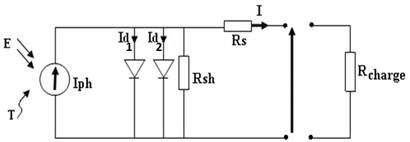Fig. 1. Equivalent model of two diode.

Using law of Kirchhoff’s, the total output current from the solar cell is given by :Where I is the net output current of the solar cell Iph is the net photo generated current from the solar cell, Id1,2 is the diodes current flowing through the diode and IP is the current through the resistances. Expanding the current equation with its internal parameter it becomes:The two diodes model consist two saturation current i.e. Is1 and Is2.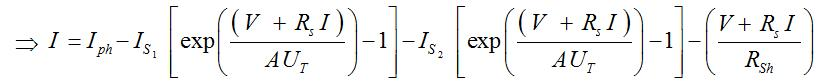Where Iph is the light generated current, Rsh is the shunt resistance, Rs is the series resistance, A is the diode ideality factor. These cell parameters control the I–V characteristics of a cell at any given intensity of illumination and cell temperature and thus determine the values of the performance parameters: the short circuit current, open circuit voltage and curve factor. As the intensity of illumination changes the values of performance parameters change significantly. The dependence of performance parameters on illumination intensity can get affected if the values of the cell parameters Rsh, Rs, A and Is themselves change with illumination intensity.

3. Results and Discussion

Fig 2 shows the temperature effect on (I-V) characteristics of monocrystalline silicon solar cell. In constant irradiation the open circuit voltage VOC decreases with temperature. High temperature lowers the VOC and short circuit ICC current increases with temperature. This increase is much less than the voltage drops. The influence of temperature on the ICC can be neglected in the majority of cases.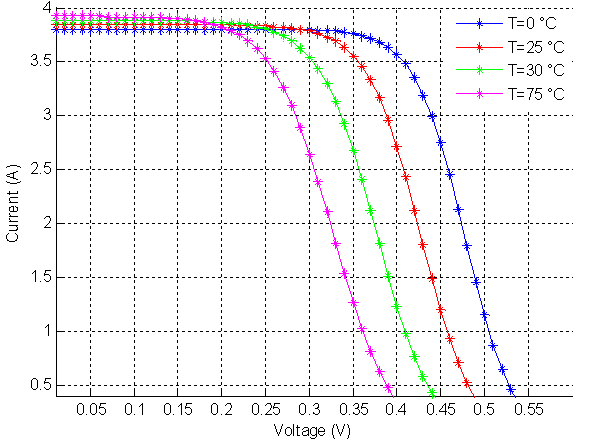Fig. 2. (I-V) characteristics of monocrystalline silicon solar cell: Temperatures effect.

Fig. 3 shows the RSh effect on (I-V) characteristics of monocrystalline silicon results in a minor reduction of the open circuit voltage, and an increase in the slope of the (I-V) of cell in the area corresponding to operation as a power supply. This is because it should subtract the photocurrent, besides the diode forward current, an additional current which varies linearly with the developed tension.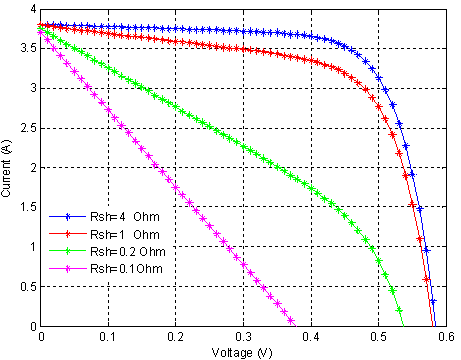Fig. 3. (I-V) characteristics of monocrystalline silicon solar cell: RSh effect.

Fig. 4 shows the RS influence on the (I -V) characteristic of monocrystalline silicon solar cell. The series resistance is the slope of the characteristic in the area or the photodiode behaves like a voltage generator. It does not modify the open circuit voltage VOC and when it is high and decreases the value of the short-circuit current.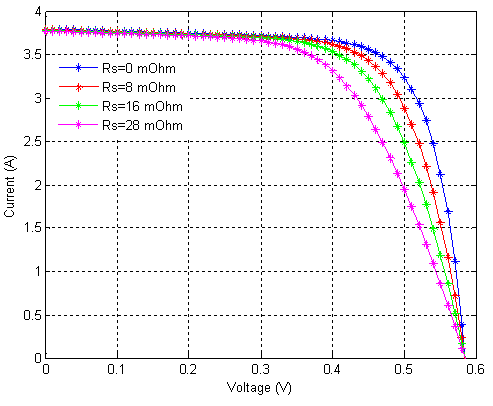Fig. 4. (I-V) characteristics of monocrystalline silicon solar cell: RS effect.Fig. 5. (I-V) characteristics of monocrystalline silicon solar cell: A effect.

Fig. 5 shows the A influence on the (I -V) characteristic of monocrystalline silicon solar cell.

A perfect match to theory is indicated when n = 1. The effect of changing ideality factor independently of all other parameters is shown for a monocrystalline silicon solar cell in the (I -V) curves displayed in the Fig. 5.

Most solar cells, which are quite large compared to conventional diodes, well approximate an infinite plane and will usually exhibit near-ideal behavior (n ≈ 1).

4. Conclusion

We have investigated the effect of temperature, series resistance and shunt resistance on the current-voltage (I-V) characteristics simulated in Matlab / Simulink. From the results obtained, it can be concluded that the VOC decreases with temperature, RS does not modify the open circuit voltage and the RSh effect that a minor reduction of the VOC. Temperature impacts significantly on open circuit voltage but almost has no effect on short circuit current.

References

1. V. Avrutin, N. Izyumskaya, H. Morkoc, "Amorphous and micromorph Si solar cells: current status and outlook", Turk Journal of Physics 2014, 38: 526 -542
2. F. Ghani, G. Rosengarten, M. Duke, J.K. Carson, "The numerical calculation of single-diode solar-cell modelling parameters", Renewable Energy 2014, 72: 105-112
3. A. El-Shaer, , M. T. Y. Tadros, M. A. Khalifa, "Effect of Light intensity and Temperature on Crystalline", The International Journal of Emerging Technology and Advanced Engineering 2014, 4: 311-318
4. E. Cuce, P. Cuce, T. Bali "An experimental analysis of illumination intensity and temperature dependency of photovoltaic cell parameters", Applied Energy 2013, 111: 374-382
5. M. Chegaar, A. Hamzaoui, A. Namoda, P. Petit, M. Aillerie and A. Herguth "Effect of Illumination Intensity on Solar Cells Parameters", Energy Procedia 2013, 36:722-729
6. O. Breitenstein "An Alternative One-diode Model for Illuminated Solar Cells", Energy Procedia 2014, 55: 30-37
7. E. Radziemska "The effect of temperature on the power drop in crystalline silicon solar cells", Renew Energy 2003, 28:1-12.
8. R. Foster, M. Ghassemi, and A. Cota, "Solar Energy: Renewable Energy and the Environment", CRC Press Taylor & FrancisGroup, Boca Raton, Fla, USA, 2010
9. S. Ndoye, I. Ly, F. Barro, O. Lemrabott, G. Sissoko"Modélisation et simulation sous Matlab/Simulink de la chaine d’alimentation d’une station relais de télécommunications en énergie solaire photovoltaïque dans une zone isolée du réseau électrique", J. Sci., 2009, 9: 45-55
10. F. Khan, S.N. Singh, M. Husain "Effect of illumination intensity on cell parameters of a silicon solar cell", Solar Energy Materials & Solar cells 2010, 94: 1473-1476
11. D. Rodriguez, P. Horley, J. Hernandez, V. Vorobiev, N. Gorley, "Photovoltaic solar cells performance at elevated temperatures", Solar Energy 2005, 78: 243-250.
12. T. Meng, C. Zhang, C. Liu, S. Meng, "Experimental Study of Light Intensity on I-V Characteristic of Single Crystalline Silicon Solar Cell", Advanced Materials Research 2012, 418-420: 725-1728.
13. E. Skoplaki, A. PalyvosJ "On the temperature dependence of photovoltaic module electrical performance: a review of efficiency/power correlations", Solar Energy Materials & Solar cells 2009, 83: 614-24
14. K. Ishaque, Z. Salam, H. Taheri, A. Shamsudin "Simple, fast and accurate two-diode model for photovoltaic modules", Solar Energy 2011, 85: 1768-1779
15. U. Stutenbaeumer, B. Mesfin, "Equivalent model of monocrystalline, polycrystalline and amorphous silicon solar cells", Renewable Energy 1999, 18: 501-512

 Contents 1. 2. 3. 4.
Article ToolsAbstractPDF(262K)